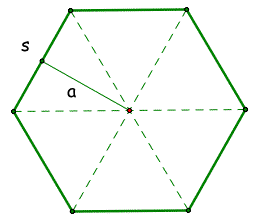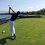# Proof for area of any Regular Polygon 2!Here is the link to my previous proof for the area of any quadrilateral being $\frac{ap}{2}$ where a is the apothem and p is the perimeter. These two notes are quite closely related and the other note helps you to get a better understanding of this note but it is not at all necessary.

In this note, I will prove that the area of any polygon is $\dfrac{1}{4} na^2\cot(\dfrac{\pi}{n})$ where n is the number of sides and a is the side length.

We start by drawing lines to each of the vertices of the polygon. Each of the angles will be congruent and of course add up to 360 deg. Thus since there are n angles (n being the number of sides), each angle is $\frac{360}{n}$.

Next, we draw the perpendicular bisectors of each side. Because each of the interior triangles is isosceles, the bisector will bisect both the angle and the side length. This makes the base of each "half" triangle $\frac{a}{2}$ and the angle closest to the polygon's in-center $\frac{180}{n}\Rightarrow \frac{\pi}{n}$).

Next, we find the height of the triangle (which is equivalent to the apothem. In this case, because we are given the angle closest to the incenter of the polygon and the corresponding opposite side length $(\frac{a}{2})$, we must multiply the base by $\cot\dfrac{\pi}{n}$. By doing this, we are left with the height since cot represents (in this case) the $\frac{adjacent}{base}$.

Then, we get the area of each triangle $\dfrac{b\times h}{2}\Rightarrow \dfrac{a\times (\dfrac{a}{2} \times (\cot\dfrac{\pi}{n}))}{2}\Rightarrow \dfrac{1}{4} a^2\cot(\dfrac{\pi}{n})$.

Finally, because there are as many triangles as there are sides, we multiply our formula by n (the number of sides and are left with $\boxed{\dfrac{1}{4} na^2\cot(\dfrac{\pi}{n})}$.Note by Trevor Arashiro
6 years, 10 months ago

This discussion board is a place to discuss our Daily Challenges and the math and science related to those challenges. Explanations are more than just a solution — they should explain the steps and thinking strategies that you used to obtain the solution. Comments should further the discussion of math and science.

When posting on Brilliant:

• Use the emojis to react to an explanation, whether you're congratulating a job well done , or just really confused .
• Ask specific questions about the challenge or the steps in somebody's explanation. Well-posed questions can add a lot to the discussion, but posting "I don't understand!" doesn't help anyone.
• Try to contribute something new to the discussion, whether it is an extension, generalization or other idea related to the challenge.

MarkdownAppears as
*italics* or _italics_ italics
**bold** or __bold__ bold
- bulleted- list
• bulleted
• list
1. numbered2. list
1. numbered
2. list
Note: you must add a full line of space before and after lists for them to show up correctly
paragraph 1paragraph 2

paragraph 1

paragraph 2

[example link](https://brilliant.org)example link
> This is a quote
This is a quote
    # I indented these lines
# 4 spaces, and now they show
# up as a code block.

print "hello world"
# I indented these lines
# 4 spaces, and now they show
# up as a code block.

print "hello world"
MathAppears as
Remember to wrap math in $$ ... $$ or $ ... $ to ensure proper formatting.
2 \times 3 $2 \times 3$
2^{34} $2^{34}$
a_{i-1} $a_{i-1}$
\frac{2}{3} $\frac{2}{3}$
\sqrt{2} $\sqrt{2}$
\sum_{i=1}^3 $\sum_{i=1}^3$
\sin \theta $\sin \theta$
\boxed{123} $\boxed{123}$

Sort by:

Good work. (y)

- 6 years, 10 months ago

Write a comment or ask a question...

- 6 years, 10 months ago

Haha. Good use of the back ground text.

- 6 years, 10 months ago

Shouldn't the formula be n (a^2)tan(pi/n) paragraph 2 If "a" is the radius, then the area converges to pi at n=infinity

- 6 years, 10 months ago

Well, if we try a triangle of side length 6, the area is $9\sqrt3$. By your formula, it's supposedly $3(36)tan(60)=108\sqrt3$

- 6 years, 10 months ago

"a" is the radius, not the side length. If it was the side length, then your formula is correct. Your diagram made it confusing.

- 6 years, 9 months ago

absolutely brilliant well said

- 6 years, 10 months ago

Absolutely brilliant!!!!!!!! well said

- 6 years, 10 months ago

gr8 work

- 6 years, 10 months ago

This is great work. I have yet to understand trigonometry to this extent.

- 6 years, 10 months ago

awesome work.

- 6 years, 10 months ago

awesome work,

- 6 years, 10 months ago

45

- 6 years, 10 months ago

awesome!!!!!!!!! well said :)

- 6 years, 10 months ago

thanks a lot

- 6 years, 10 months ago

toooooooooo good

- 6 years, 9 months ago

you cheat !!!!!!!(to sumit sakarkhar

- 6 years, 9 months ago

Could we say the triangles formed are equilateral? For example a hexagon would have 6 equilateral triangles area would be 6a^2root(3)/4 ??

- 6 years, 9 months ago